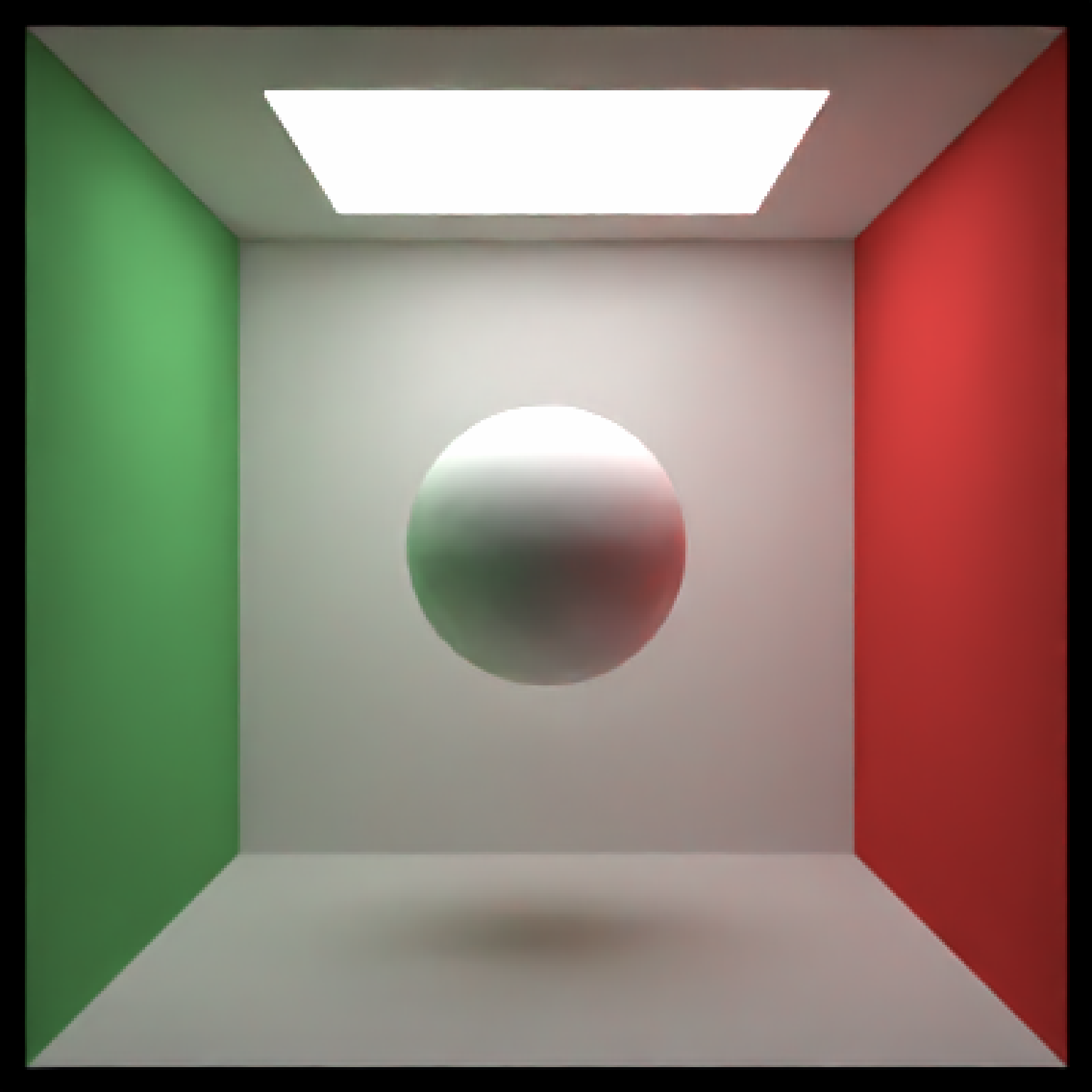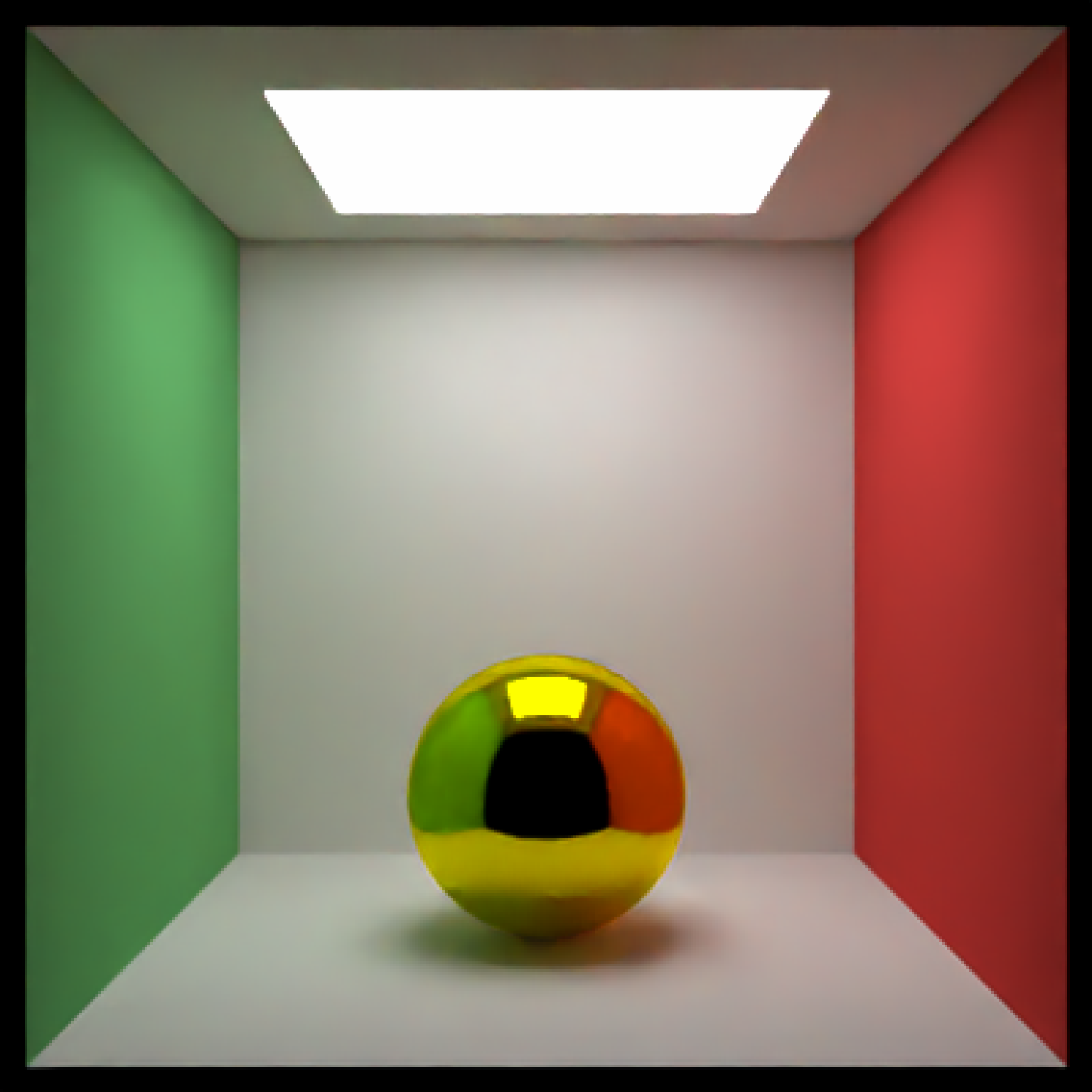Sphere Object

sphere(x = 0, y = 0, z = 0, radius = 1, material = lambertian(),
angle = c(0, 0, 0), order_rotation = c(1, 2, 3), velocity = c(0, 0,
0), flipped = FALSE)

## Arguments

x Default 0. x-coordinate of the center of the sphere. Default 0. y-coordinate of the center of the sphere. Default 0. z-coordinate of the center of the sphere. Default 1. Radius of the sphere. Default lambertian.The material, called from one of the material functions lambertian, metal, or dielectric. Default c(0,0,0). Angle of rotation around the x, y, and z axes, applied in the order specified in order_rotation. Default c(1,2,3). The order to apply the rotations, referring to "x", "y", and "z". Default c(0,0,0). Velocity of the sphere, used for motion blur. Default FALSE. Whether to flip the normals.

## Value

Single row of a tibble describing the sphere in the scene.

## Examples

#Generate a sphere in the cornell box.
generate_cornell() %>%
render_scene(lookfrom = c(278,278,-800) ,lookat = c(278,278,0), fov = 40, ambient_light=FALSE)render_scene(lookfrom = c(278,278,-800) ,lookat = c(278,278,0), fov = 40, ambient_light=FALSE)render_scene(lookfrom = c(278,278,-800) ,lookat = c(278,278,0), fov = 40, ambient_light=FALSE)